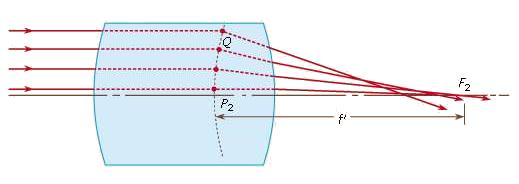Courses

# The Gauss theory of lenses Physics Notes | EduRev

## Physics : The Gauss theory of lenses Physics Notes | EduRev

The document The Gauss theory of lenses Physics Notes | EduRev is a part of the Physics Course Basic Physics for IIT JAM.
All you need of Physics at this link: Physics

In 1841 Gauss published a now famous treatise on optics in which he demonstrated that, so far as paraxial rays are concerned, a lens of any degree of complexity can be replaced by two principal, or nodal, points and two focal points, the distances from the principal points to their respective focal points being the focal lengths of the lens, and, furthermore, that the two focal lengths are equal to one another when the refractive indices of object and image spaces are equal, as when a lens is used in air.

The principal and focal points may be defined as follows: Figure shows a lens system of any construction, with a bundle of rays entering from the left in a direction parallel to the lens axis. After refraction by the lens each ray will cross the axis at some point, and the entering and emerging portions of each ray are then extended until they intersect at a point such as Q. The locus of all the points Q is a surface of revolution about the lens axis known as the equivalent refracting locus of the lens. The point where this locus crosses the axis is called the principal point, P2, and the central portion of the locus in the neighbourhood of the axis, which is virtually a plane perpendicular to the axis, is called the principal plane. The point where the emerging paraxial ray crosses the axis is called the focal point F2, the distance from P2 to F2 being the (posterior) focal length f′. A similar situation exists for a parallel beam of light entering from the right, giving the anterior principal point P1, the anterior focal point F1, and the front focal length f. For a lens in air it can be shown that the two focal lengths are equal in magnitude but opposite in direction—i.e., if F2 is to the right of P2, then F1 must lie to the left of P1, as in the case of an ordinary positive lens (one that gives a real image). In a negative lens (one that gives a virtual image), F2 lies to the left of P2, and the posterior focal length f′ is negative.Figure 5: The Gauss theory (see text).

The relation between the distances of object and image from a lens can be easily stated if the positions of the two principal points and the two focal points are known. (In using these expressions, distances are considered positive or negative depending on whether they are measured to the right or to the left from their respective origins.) For a lens in air: (a) If the conjugate distances measured from the respective focal points are x and x′, and if m is the image magnification (height of image divided by height of object), then m = -x′/f′ = f′/x and xx′ = −f2. (b) If the conjugate distances measured from the respective principal points are p and p′ and if m is the image magnification, then m = p′/p and 1/p′ = 1/p + 1/f′. The Lagrange equation requires modification for a distant object because in that case the object height h is infinite, and the slope angle u is zero. If the off-axis distance h is divided by the object distance L, and u is multiplied by L, equation (7) becomes h′ = (n/n′)f′ϕ, in which ϕ is the angle in radians subtended by the distant object at the lens. This formula provides a means for defining focal length and for measuring the focal length of an unknown lens.

h'n'u = hnu, (7)

Offer running on EduRev: Apply code STAYHOME200 to get INR 200 off on our premium plan EduRev Infinity!

,

,

,

,

,

,

,

,

,

,

,

,

,

,

,

,

,

,

,

,

,

;